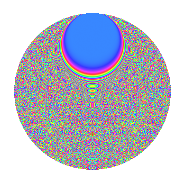# Properties

 Label 2541.2.btLevel 2541 Weight 2 Character orbit bt Rep. character $$\chi_{2541}(89,\cdot)$$ Character field $$\Q(\zeta_{66})$$ Dimension 6960 Sturm bound 704

# Related objects

## Defining parameters

 Level: $$N$$ = $$2541 = 3 \cdot 7 \cdot 11^{2}$$ Weight: $$k$$ = $$2$$ Character orbit: $$[\chi]$$ = 2541.bt (of order $$66$$ and degree $$20$$) Character conductor: $$\operatorname{cond}(\chi)$$ = $$2541$$ Character field: $$\Q(\zeta_{66})$$ Sturm bound: $$704$$

## Dimensions

The following table gives the dimensions of various subspaces of $$M_{2}(2541, [\chi])$$.

Total New Old
Modular forms 7120 7120 0
Cusp forms 6960 6960 0
Eisenstein series 160 160 0

## Trace form

 $$6960q - 60q^{3} - 362q^{4} - 40q^{7} - 24q^{9} + O(q^{10})$$ $$6960q - 60q^{3} - 362q^{4} - 40q^{7} - 24q^{9} - 15q^{12} - 18q^{15} + 326q^{16} - 37q^{18} - 54q^{19} - 41q^{21} - 176q^{22} + 30q^{24} + 310q^{25} - 20q^{28} - 57q^{30} - 42q^{31} - 33q^{33} + 12q^{36} - 26q^{37} - 4q^{39} - 192q^{40} + 57q^{42} - 104q^{43} + 21q^{45} - 26q^{46} + 40q^{49} - 17q^{51} - 264q^{52} + 297q^{54} - 102q^{57} + 74q^{58} - 64q^{60} - 54q^{61} - 6q^{63} + 240q^{64} - 102q^{66} + 14q^{67} + 64q^{70} + 41q^{72} - 6q^{73} - 75q^{75} - 54q^{78} - 18q^{79} - 48q^{81} + 42q^{82} - 51q^{84} + 168q^{85} + 48q^{87} - 10q^{88} - 84q^{91} - 28q^{93} - 180q^{94} - 15q^{96} - 242q^{99} + O(q^{100})$$

## Decomposition of $$S_{2}^{\mathrm{new}}(2541, [\chi])$$ into newform subspaces

The newforms in this space have not yet been added to the LMFDB.

## Hecke Characteristic Polynomials

There are no characteristic polynomials of Hecke operators in the database